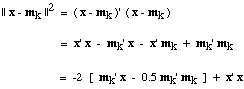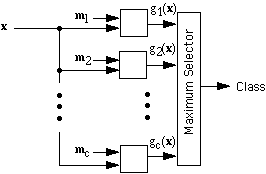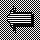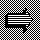# Linear Discriminants

Recall that when we use a minimum-distance classifier to classify a feature vector x, we measure the distance from x to the templates m1, m2, ..., mc and assign x to the class of the nearest template. Using the inner product to express the Euclidean distance from x to mk, we can writeNotice that the x'x term is the same for every class, i.e., for every k. To find the template mk that minimizes || x - mk ||, it is sufficient to find the mk that maximizes the bracketed expression, mk' x - 0.5 mk' mk. Let us define the linear discriminant function g(x) by

g(x) = m' x - 0.5 || m ||2 .

Then we can say that a minimum-Euclidean-distance clasifier classifies an input feature vector x by computing c linear discriminant functions g1(x), g2(x), ... , gc(x) and assigning x to the class corresponding to the maximum discriminant function. We can also think of the linear discriminant functions as measuring the correlation between x and mk, with the addition of a correction for the "template energy" represented by || mk ||2. With this correction included, a minimum-Euclidean-distance classifier is equivalent to a maximum-correlation classifier.Back to Inner Prod.On to BoundariesUp to Simple Classifiers